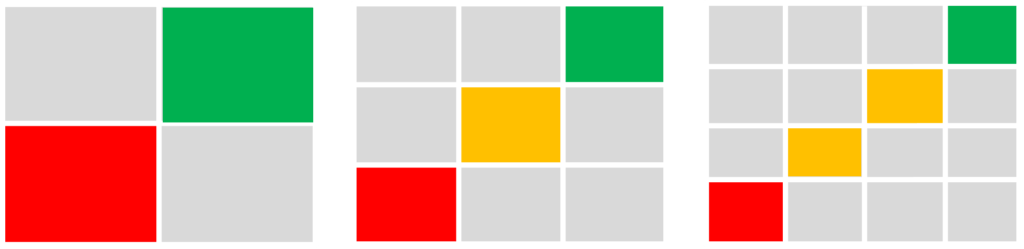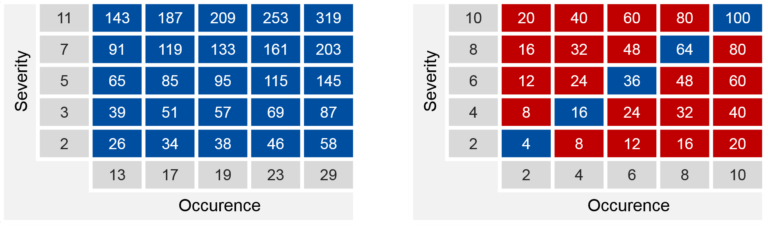## even levels - evil levelsIn most projects, risk management is understood as conducting the exercise of failure-mode and effects analysis, short FMEA. But with this, there comes a risk. Do you use even levels for the severity (impact), occurrence (likelihood), or detectability rates? If so, you might be exposed to an unknown risk – the “evil levels bias risk”.

### FMEA is biased

In most cases when FMEAs are conducted, a risk level map is generated based on rate levels to render the FMEA quantitative. Choosing these levels is crucial for an unbiased FMEA experience and thus, unbiased risk reduction measures.

“Which levels for rating should we use in a FMEA?”

Robert

Medical Device Company

We often get the question form clients “Which levels for rating should we use in a FMEA?” –  the answer to this is quite simple, but hold on, no spoiler alert yet. The issue with a bias in the rating levels stems from the fact that risk levels should be chosen in such a way as to let the user decide on whether a risk should be further mitigated or can be accepted by the organization.

The risks resulting from such analysis are often rated by numbers transformed by multiplication into a risk number. This risk number is then used to assess and decide on acceptability, as given in ISO 14971 or ICH Q9. But, there is an intrinsic difference between a risk with a risk number of 12 and the other risk with the same risk number of 12 because they might root from different severity levels and different occurrence levels.

The bias in multiplication comes from losing information of the single factors. For example, a value of 12 can root from a multiplication of the factors 2 and 6, or 6 and 2, or 3 and 4 or 4 and 3. And here is the first step towards an unbiased risk level matrix. Thus, a severity of 6 might have more impact on an organization than a severity of 2. Likewise, the occurrence rates of 2 and 6 cipher for different needed measures to reduce the risk.

### How many levels should we use?There is no distinct requirement for the number of levels but most of the industry uses a 5-level approach. At least, an odd number of levels should be used in order to have at least an acceptable level, a further to mitigate level and an unacceptable level.

### Use only odd numbers for the rate levels:

Again, the multiplication eats up information or so-called degrees of freedom and thus, smart rating levels should be odd to reduce the bias and information loss after multiplication. However, beware from using the number 1 as a rating level as this would add itself to any level and thus increase bias again.

### Use only prime numbers for the rate levels:

What happens therefore if we use only odd numbers as the rating levels? Well, we end up with matrix of reduced bias but there is more to it to reduce the bias to the minimum and have absolute numbers to decipher for specific severity, occurrence and detection levels. The trick to the game is to use only prime numbers. The beauty of prime numbers is that these are only dividable by itself and 1. As we have excluded 1 already as one of the “evil numbers” adding bias, we end up with the prime numbers only.

### Use different prime numbers per rating levelHaving reduced the rating levels to only prime numbers has greatly reduced the bias in the resulting value derived by multiplication. However, to yield distinct values to decipher easily for the severity, occurrence and detection level, the rating levels must be different per rating. With this, the rating levels for severity, occurrence and detection might take use of the following values:

2, 3 ,5 , 7, 11

#### Occurence

13, 17, 19, 23, 29

#### detectability

31, 37, 41, 43, 47

The resulting matrices are unbiased and let you and your teams derive for specific values of severity, occurrence and detection levels. With this, you stop the bias in your risk management and have continuously the correct picture of the risks you are accepting or need further mitigation.

Need help on risk management? Contact us to discuss your needs and how we can help to bring your risk management file back to an unbiased level.